# 5. Let the joint probability density function of X and Y be given by, f(x,y) =...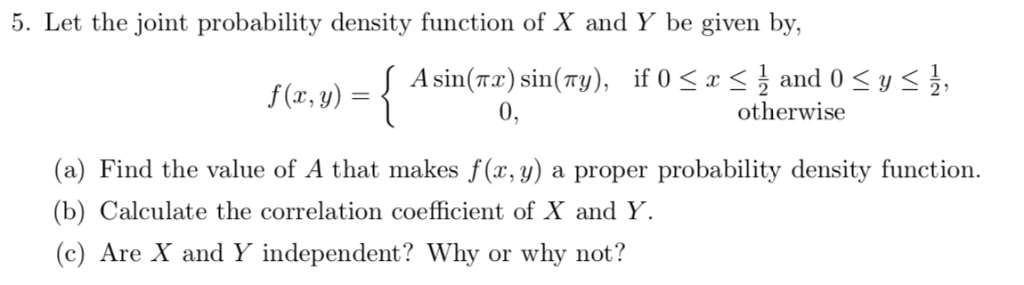5. Let the joint probability density function of X and Y be given by, f(x,y) = 0 otherwise (a) Find the value of A that makes f (x, y) a proper probability density function (b) Calculate the correlation coefficient of X and Y. (c) Are X and Y independent? Why or why not?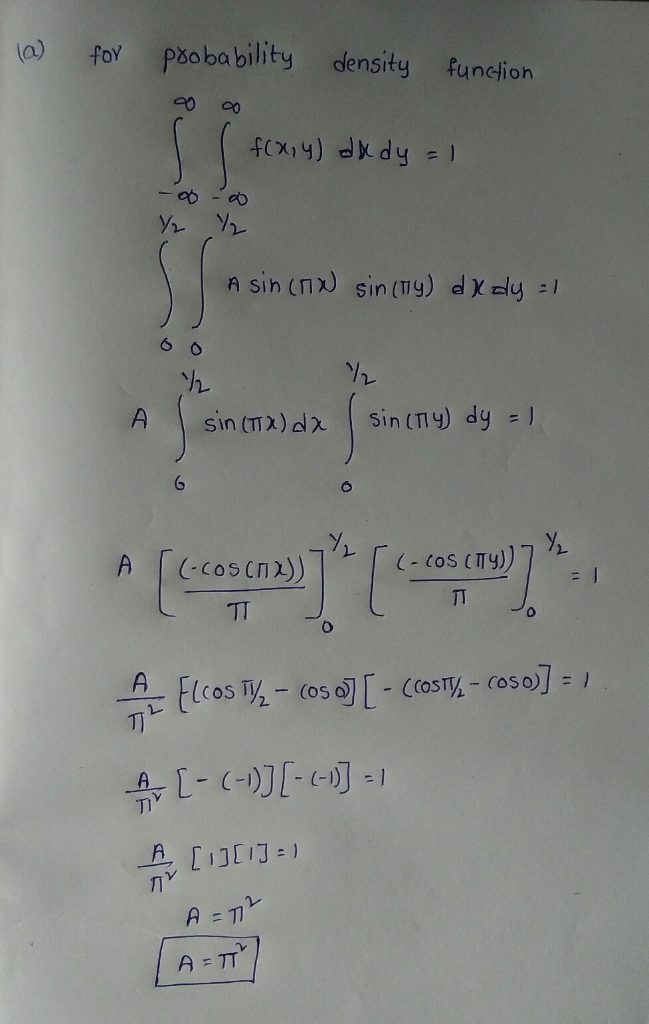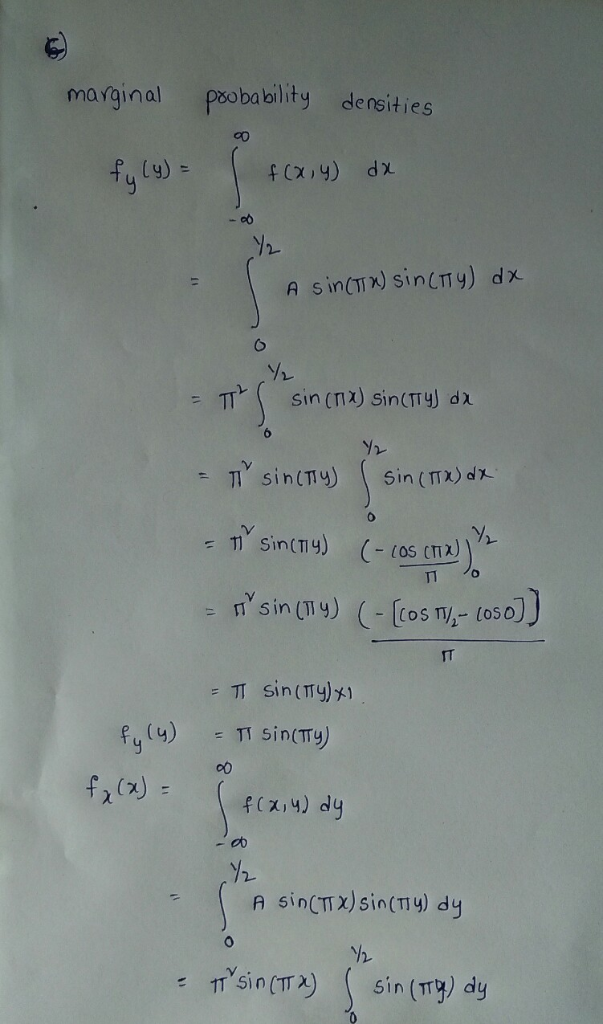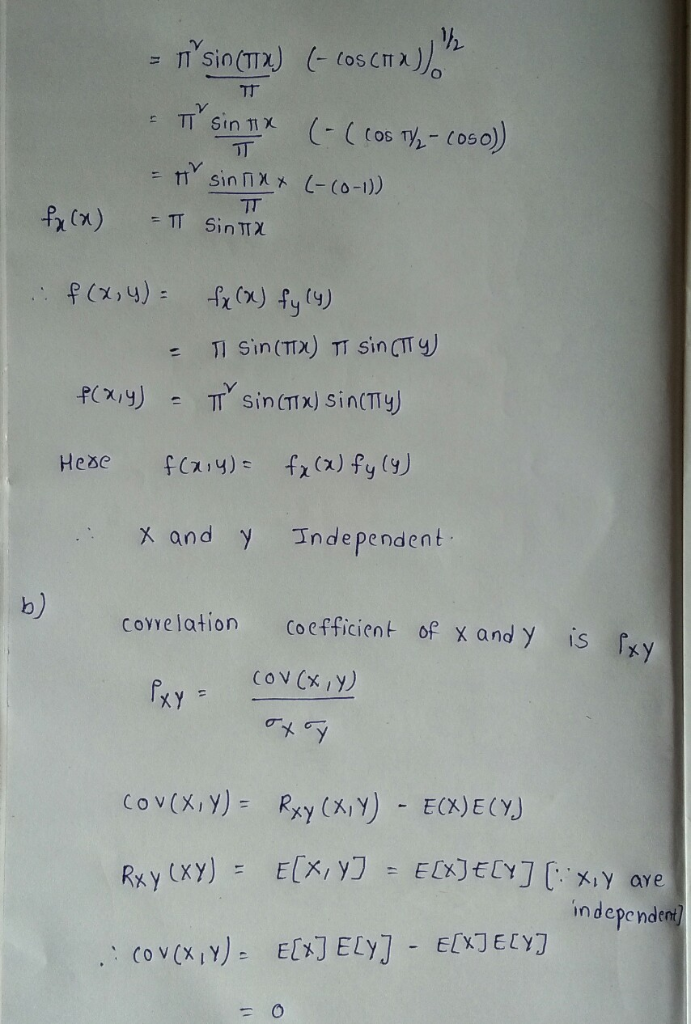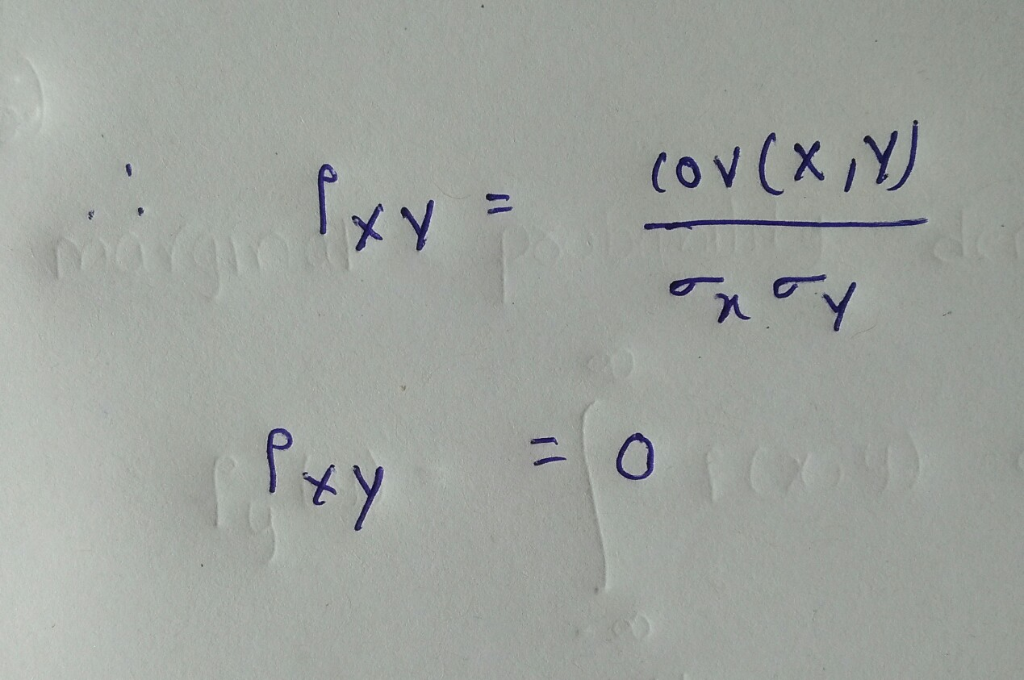#### Earn Coin

Coins can be redeemed for fabulous gifts.

Similar Homework Help Questions
• ### 5. Let X and Y have joint probability density function of the form Skxy if 0...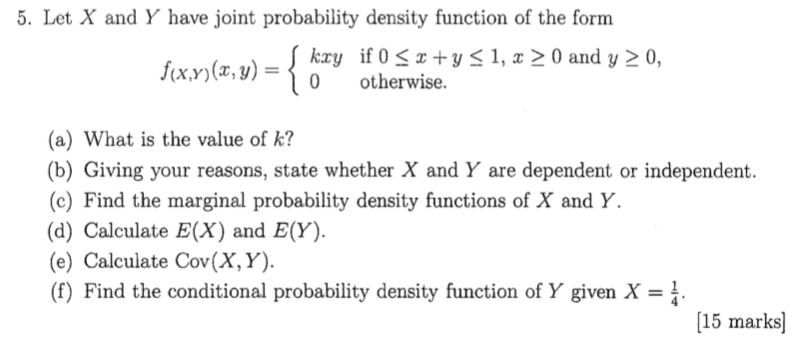5. Let X and Y have joint probability density function of the form Skxy if 0 < x +y < 1, x > 0 and y > 0, f(x,y)(, y) = { 0 otherwise. (a) What is the value of k? (b) Giving your reasons, state whether X and Y are dependent or independent. (c) Find the marginal probability density functions of X and Y. (d) Calculate E(X) and E(Y). (e) Calculate Cov(X,Y). (f) Find the conditional probability density function...

• ### 2. Let X and Y be continuous random variables with joint probability density function fx,y(x,y) 0, otherwise (a) Compute the value of k that will make f(x, y) a legitimate joint probability density f...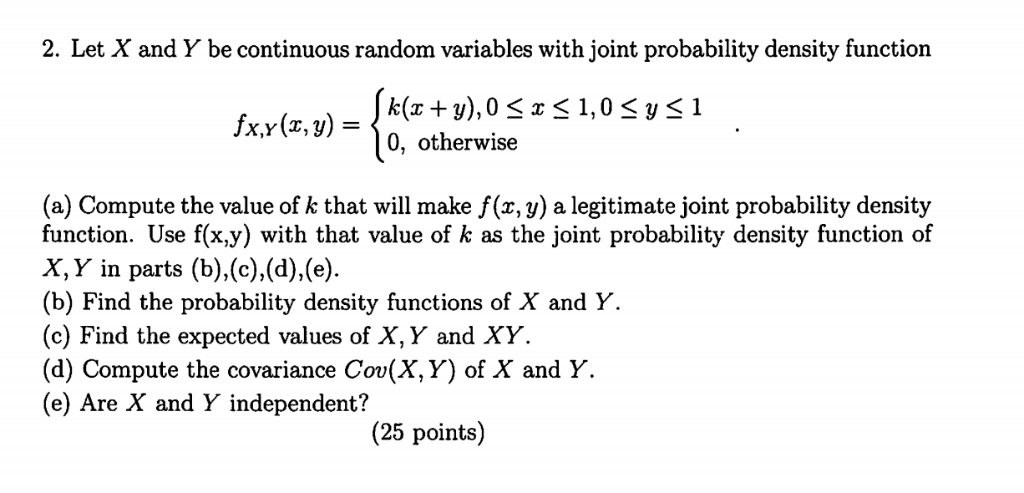2. Let X and Y be continuous random variables with joint probability density function fx,y(x,y) 0, otherwise (a) Compute the value of k that will make f(x, y) a legitimate joint probability density function. Use f(x.y) with that value of k as the joint probability density function of X, Y in parts (b),(c).(d),(e (b) Find the probability density functions of X and Y. (c) Find the expected values of X, Y and XY (d) Compute the covariance Cov(X,Y) of X...

• ### 1. Let X and Y have the joint density function given by zob to todos f(x,...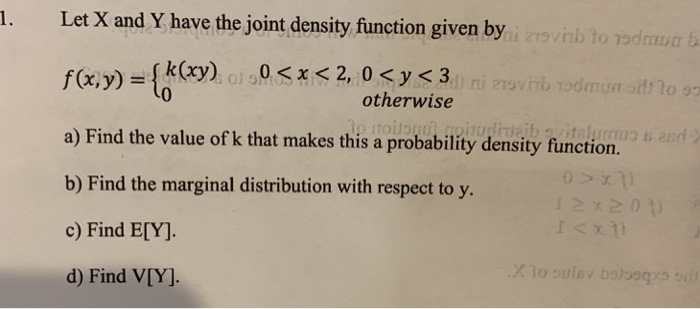1. Let X and Y have the joint density function given by zob to todos f(x, y) = {kxy) of 50<x< 2, 0 <y<3.) i 279VHb yodmu : 1093 otherwise a) Find the value of k that makes this a probability density function. TO B 250 b) Find the marginal distribution with respect to y. 0x11 sono c) Find E[Y] d) Find V[Y]. X10 sulay boso 50

• ### 2. Let the joint probability density function of (X, Y) be given by {ay otherwise. 1 and 0 < y < 2, f(z,y) (a) [6 pts] Determine if X and Y are independent. (b) [6 pts] Find P{X+Y <1)...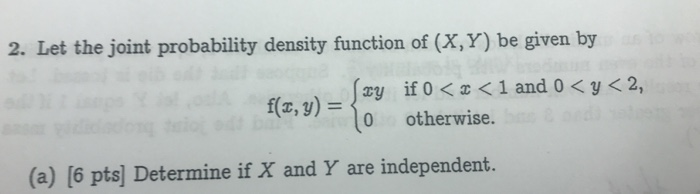2. Let the joint probability density function of (X, Y) be given by {ay otherwise. 1 and 0 < y < 2, f(z,y) (a) [6 pts] Determine if X and Y are independent. (b) [6 pts] Find P{X+Y <1) B( (c) [6 pts) Find 2. Let the joint probability density function of (X, Y) be given by {ay otherwise. 1 and 0

• ### 5. Let the joint cumulative density function of random variables X and Y be given by...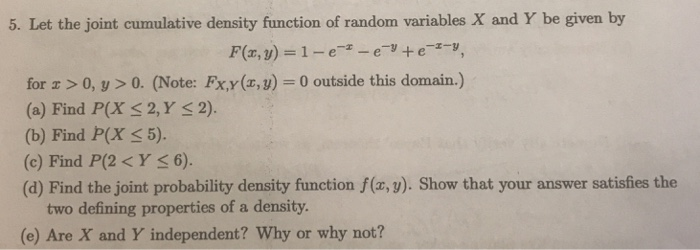5. Let the joint cumulative density function of random variables X and Y be given by for z 0, y >0. (Note: Fxy(x, y)-0 outside this domain.) (a) Find P(X S2,Y (b) Find P(X5). (c) Find P(2 <Y s6). (d) Find the joint probability density function f(x, y). Show that your answer satisfies the S 2). two defining properties of a density. (e) Are X and Y independent? Why or why not?

• ### 2. Suppose that (X,Y) has the following joint probability density function: f(x,y) = C if -1...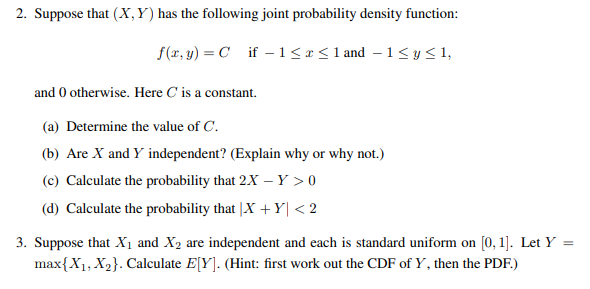2. Suppose that (X,Y) has the following joint probability density function: f(x,y) = C if -1 <r< 1 and -1 <y<1, and 0 otherwise. Here is a constant. (a) Determine the value of C. (b) Are X and Y independent? (Explain why or why not.) (c) Calculate the probability that 2X - Y > 0 (d) Calculate the probability that |X+Y| < 2 3. Suppose that X1 and X2 are independent and each is standard uniform on (0,1]. Let Y...

• ### 7. The random variables X and Y have joint probability density function f given by 1...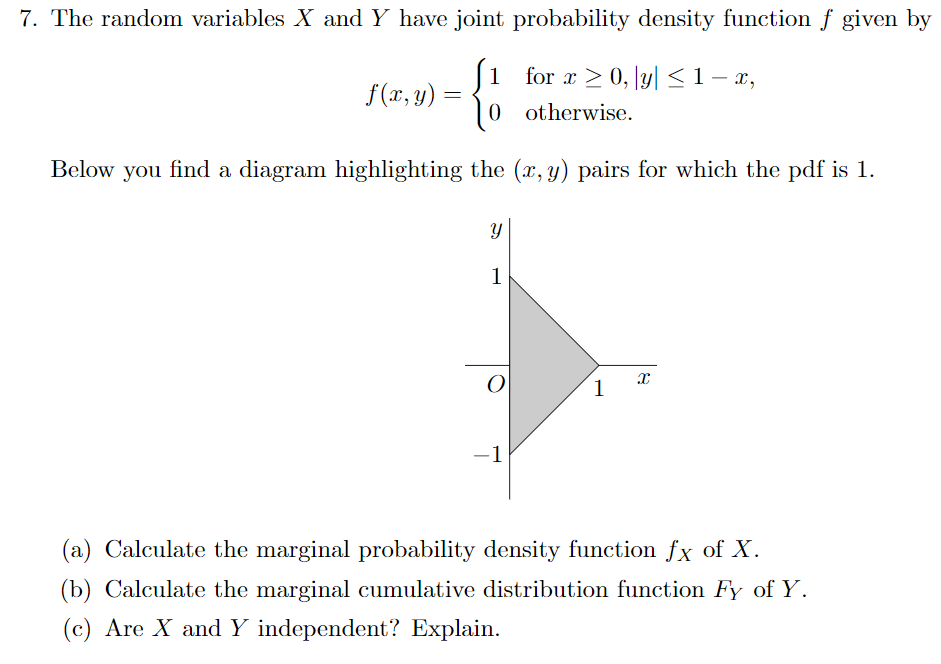7. The random variables X and Y have joint probability density function f given by 1 for x > 0, |y| 0 otherwise. 1-x, Below you find a diagram highlighting the (r, y) pairs for which the pdf is 1 (a) Calculate the marginal probability density function fx of X (b) Calculate the marginal cumulative distribution function Fy of Y (c) Are X and Y independent? Explain.

• ### 7. The random variables X and Y have joint probability density function f given by 1...7. The random variables X and Y have joint probability density function f given by 1 for x > 0, |y| 0 otherwise. 1-x, Below you find a diagram highlighting the (r, y) pairs for which the pdf is 1 (a) Calculate the marginal probability density function fx of X (b) Calculate the marginal cumulative distribution function Fy of Y (c) Are X and Y independent? Explain.

• ### (pts) 1. The joint probability density of X and Y is given by . 0<x<1 and...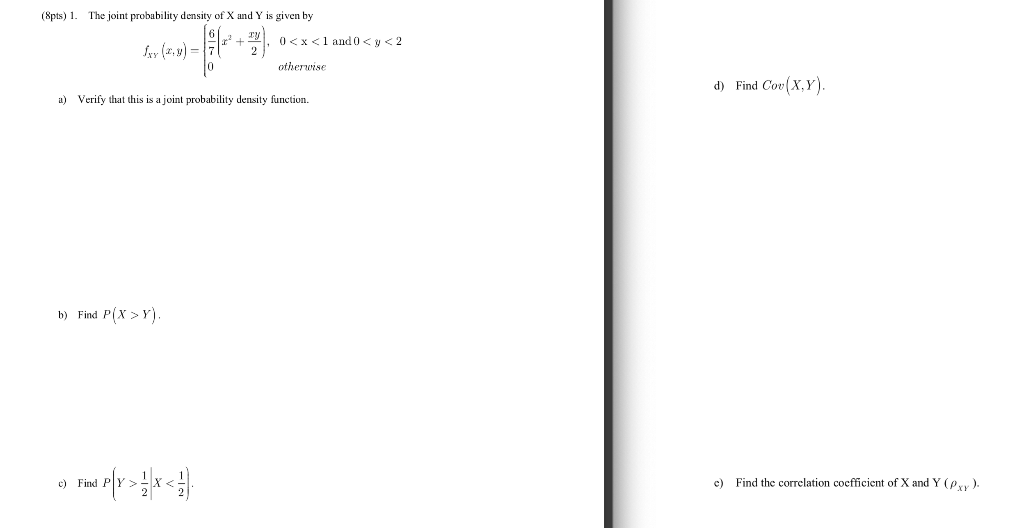(pts) 1. The joint probability density of X and Y is given by . 0<x<1 and 0 <y<2 otherwise d) Find Cov(X,Y). a) Verify that this is a joint probability density function. b) Find P(x >Y). ) Find Pſy>*<51 c) Find the correlation coefficient of X and Y (Pxy).

• ### Let the random variables X, Y with joint probability density function (pdf) fxy(z, y) = cry,...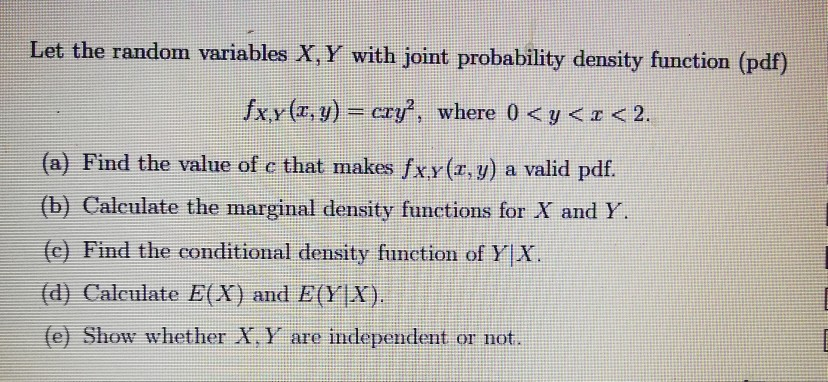Let the random variables X, Y with joint probability density function (pdf) fxy(z, y) = cry, where 0 < y < z < 2. (a) Find the value of c that makes fx.y (a, y) a valid pdf. (b) Calculate the marginal density functions for X and Y (c) Find the conditional density function of Y X (d) Calculate E(X) and EYIX) (e Show whether X. Y are independent or not.